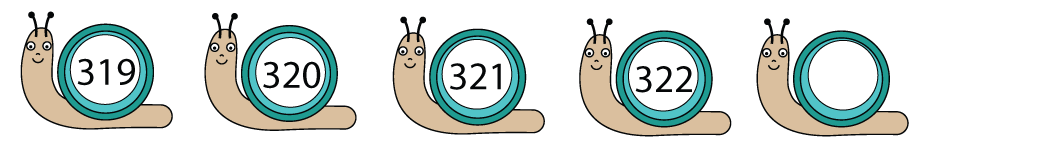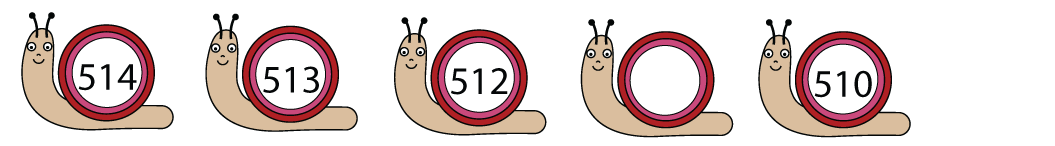A sequence is a list of numbers that are linked by a rule. If we find the rule, we can work out the next numbers in the sequence.

Look at this example:The pattern here is to add 1 to each number.

322 + 1 is 323, so the next number is 323.

Look at this next example:The pattern is to take 1 away from each number.

512 − 1 is 511, so the missing number is 511.